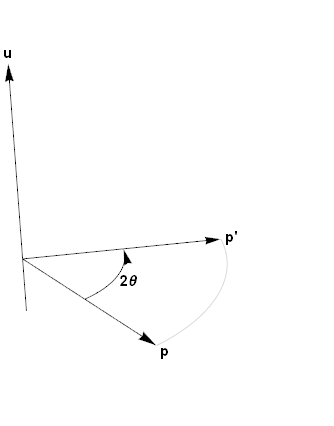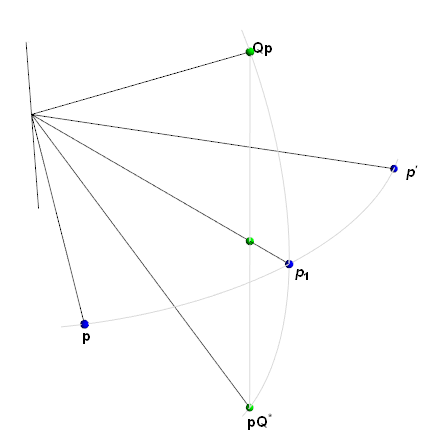Currently the Wikipedia page on quaternion rotations gives you this helpful figure to explain $Q\mathbf{p}$ portion of $\eqref{xform}$ when the rotation angle is $\frac{\pi}{3}$. I will try to do a little better.Given a non-zero quaternion $Q$ we can rotate a 3D coordinate $\mathbf{p}$ with the similarity transform:

$\begin{equation} \label{eq:sxform} \mathbf{p}' = Q~\mathbf{p}~Q^{-1} \end{equation}$

and by restricting ourselved to unit quaternions this reduces to:

$\begin{eqnarray} \mathbf{p}' & = & Q~\mathbf{p}~Q^* \label{xform} \\ & = & \left(Q\mathbf{p}\right)Q^* \label{xforml} \\ & = & Q\left(\mathbf{p}Q^*\right) \label{xformr} \end{eqnarray}$

where $\eqref{xforml}$ and $\eqref{xformr}$ are simply stating that quaternion products are associative.

if we have unit quaternion: $Q = \cos\left(\theta\right)+\sin\left(\theta\right)~\mathbf{u}$, which represents a $2\theta$ rotation about $\mathbf{u}$, then we have:.

### The rotation

Still here? Okay instead of using the traditional basis set, define three unit bivectors 1:

$\begin{eqnarray*} \mathbf{x} & = & \left(1,0,0\right) \\ \mathbf{y} & = & \left(0,1,0\right) \\ \mathbf{z} & = & \left(0,0,1\right) \end{eqnarray*}$

All rotations are mathematically similar so we can choose an arbitrary fixed axis like “up”, which we can all agree is positive Z. To be “mathy” let’s define the set of quaterions with the following form: $a + b~\mathbf{z}$ where $a$ is a real and $b$ is a positive (or zero) real. In set-builder notation: $\newcommand{\hcomplex}{\mathbb{C}_{#1}}$

$\hcomplex{z}=\left\{ \left(a + b~\mathbf{z}\right) \in\mathbb{H}:a,b~\mathbb{\in R} \right\}$

The members of this set form a plane which spans (contains) the line-of-reals ($\mathbb{R}$) and $~\mathbf{z}$. Our rotation is member of this set:

$\begin{eqnarray} Q & = & \cos\left(\theta\right)+\sin\left(\theta\right)~\mathbf{z} \label{pclassic} \end{eqnarray}$

which represents a rotation of $2\theta$ about $\mathbf{z}$.

### The complex case and Complex is easy

The simplest case is some coordinate in $\hcomplex{z}$:

$\mathbf{p}_z = \left(0,0,c\right) = c\left(0,0,1\right) = c~\mathbf{z}$

and since the product is associative $\eqref{xform}$ can be evaluated as either $\eqref{xforml}$ or $\eqref{xformr}$. Expanding the first terms of both gives:

$\begin{eqnarray*} Q\mathbf{p}_z & = & c \left(-\sin\left(\theta\right) + \cos\left(\theta\right) \mathbf{z} \right) \\ \mathbf{p}_z Q^* & = & c \left( \sin\left(\theta\right) + \cos\left(\theta\right) \mathbf{z} \right) \end{eqnarray*}$

Note that these are rotations by $\theta$ and $-\theta$ respectively in $\hcomplex{z}$ (angles are with respect to the line-of-reals so the angle of $\mathbf{p}_z$ is $\frac{\pi}{2}$).

Completing both composes the result with the opposite rotation, which cancels out any movement ($Q\mathbf{p}_z Q^* = \mathbf{p}_z$), exactly as we would expect.

To generalize this consider a unit quaternion in $\hcomplex{z}$:

$B = \cos\left(\phi\right)+\sin\left(\phi\right)~\mathbf{z}$

then the product with Q is ($\mathbf{z}^2=-1$):

$\begin{eqnarray*} QB & = & ( \cos\left(\theta\right)+\sin\left(\theta\right)~\mathbf{z} ) ( \cos\left(\phi\right)+\sin\left(\phi\right)~\mathbf{z} ) \\ & = & \cos (\theta ) \cos (\phi )-\sin (\theta ) \sin (\phi ) + (\sin (\theta ) \cos (\phi )+\cos (\theta ) \sin (\phi ))\mathbf{z} \\ & = & \cos (\theta+\phi ) + \sin (\theta + \phi )\mathbf{z} \\ & = & BQ \end{eqnarray*}$

This whole section boils down to a more general property:

Where {1,Q}-plane spans the line-of-reals and Q which in our case is $\hcomplex{z}$ (and why I chose to name the set this way). Our product is simply the complex product and we could have immediately flipped two of the term, cancelled and called it a day:

$Q\mathbf{p}_z Q^* = QQ^*\mathbf{p}_z = \mathbf{p}_{z}QQ^* = \mathbf{p}_z$

### The orthogonal case is Complex too

Now consider a coordinate independent of $\hcomplex{z}$, which falls in the {$\mathbf{x},\mathbf{y}$}-plane:

$\begin{eqnarray*} \mathbf{p}_o & = & \left(a,b,0\right) \end{eqnarray*}$

although not needed we can factor this into a $\hcomplex{z}$ term on either the left or right:

$\begin{eqnarray*} \mathbf{p}_o & = & ( a + b~\mathbf{z} )~\mathbf{x} \\ & = & \mathbf{x}~( a - b~\mathbf{z} ) \end{eqnarray*}$

I’ll leave the factorization as “black-magic” here. The math is straight forward but pretty wordy so I’ll leave that for another day. (It’s just a boring formalization of standard vector space operations anyway) In these factorized forms we can multiply on the left (for the first) or right (for the second) by a quaternion in $\hcomplex{z}$ and the first product is simply complex like the first case above:

$\begin{eqnarray*} Q\mathbf{p}_o & = & Q ( a + b~\mathbf{z} )~\mathbf{x} \\ & = & ( \cos\left(\theta\right)+\sin\left(\theta\right)~\mathbf{z} ) ( a + b~\mathbf{z} )~\mathbf{x} \\ & = & ( a \cos ( \theta )-b \sin ( \theta ) + (a \sin ( \theta )+b \cos ( \theta ) )~\mathbf{z} )~\mathbf{x} \end{eqnarray*}$

the left term is simply a rotation by $\theta$ in $\hcomplex{z}$. Completing the product maps the result back to the {$\mathbf{x},\mathbf{y}$}-plane, so it is a rotation by $\theta$ about $\mathbf{z}$ as expected.

$\begin{eqnarray*} Q\mathbf{p}_o & = & ( a \cos ( \theta )-b \sin ( \theta ), ~ a \sin ( \theta )+b \cos ( \theta ), ~0 ) \end{eqnarray*}$

Instead of mechanically expanding the right product, instead let’s mechanically apply identities:

$\begin{eqnarray} \mathbf{p}_{o}Q^* & = & \mathbf{x} ( a - b~\mathbf{z} ) Q^* \label{s1} \\ & = & \mathbf{x}~Q^*( a - b~\mathbf{z} ) \label{s2} \\ & = & \mathbf{x} Q^*( a + b~\mathbf{z} )^* \label{s3} \\ & = & \mathbf{x} ( Q ( a + b~\mathbf{z} ) )^* \label{s4} \\ & = & Q ( a + b~\mathbf{z} )~\mathbf{x} \label{s5} \\ & = & Q\mathbf{p}_o \label{s6} \end{eqnarray}$
• second factorized version $\eqref{s1}$
• the terms are in same complex plane so they commute $\eqref{s2}$
• basic conjugation property $\eqref{s3}$
• conjugate distributes $\eqref{s4}$
• ahh…see below $\eqref{s5}$
• same as previous expansion $\eqref{s6}$

so this is also a rotation by $\theta$ about $\mathbf{z}$ as expected and the composition of both results in a total rotation of $2\theta$.

All the previous steps up to $\eqref{s5}$ are in the complex plane. This is the product with a bivector orthogonal to that plane:

$\begin{eqnarray*} \mathbf{x}(s + t~\mathbf{z})^* & = & \mathbf{x}(s - t~\mathbf{z}) \\ & = & s\mathbf{x}-t(\mathbf{x}\times\mathbf{z} - \mathbf{x}\cdot\mathbf{z}) \\ & = & s\mathbf{x}+t\mathbf{y} \\ \\ (s + t~\mathbf{z})\mathbf{x} & = & s\mathbf{x}+t(\mathbf{z}\times\mathbf{x} - \mathbf{x}\cdot\mathbf{z}) \\ & = & s\mathbf{x}+t\mathbf{y} \\ \end{eqnarray*}$

### The arbitrary coordinate case

Now consider the coordinate:

$\begin{eqnarray*} \mathbf{p} & = & \left(a,b,c\right) \\ & = & \mathbf{p}_z + \mathbf{p}_o \end{eqnarray*}$

The only thing left to be said is the right and left products both distribute over addition.

$\begin{eqnarray*} A(B+C) & = & AB+AC \\ (A+B)C & = & AC+BC \\ \end{eqnarray*}$

using both results in:

$\begin{eqnarray*} Q\mathbf{p} Q^* & = & Q ( \mathbf{p}_z + \mathbf{p}_o ) Q^* \\ & = & Q \mathbf{p}_z Q^* + Q \mathbf{p}_o Q^* \\ & = & \mathbf{p}_z + Q \mathbf{p}_o Q^* \\ & = & ( a \cos ( 2\theta )-b \sin ( 2\theta ), ~ a \sin ( 2\theta )+b \cos ( 2\theta ), ~c ) \end{eqnarray*}$

## Visualization

Let’s do a quick recap. We can think in terms of an fixed axis of rotation which is positive Z in this case. We can simply expand the left-product and right-products of $\eqref{xform}$ and we get:

$\begin{eqnarray*} Q\mathbf{p} & = & (a\cos(\theta)-b\sin(\theta), ~a\sin(\theta)+b\cos(\theta), ~c \color{blue}{\cos(\theta)})\color{blue}{-c\sin(\theta)} \\ \mathbf{p}Q^* & = & (a\cos(\theta)-b\sin(\theta), ~a\sin(\theta)+b\cos(\theta), ~c \color{blue}{\cos(\theta)})\color{blue}{+c\sin(\theta)} \end{eqnarray*}$

where the black terms are the proper rotation in 3D and the blue are the rotation in the complex plane. Each product performs a rotation of $\theta$ in the {$x,y$}-plane (the desired) and a rotation of $\pm\theta$ in the {$1,z$}-plane (complex plane). These planes are orthogonal (independent).

The coordinate of both in the orthogonal plane can expressed as the 2D coordinate:

$(a\cos(\theta)-b\sin(\theta), ~a\sin(\theta)+b\cos(\theta))$

which composes to a total rotation of $2\theta$. The 2D coordinate in the complex plane, after factoring out the scale and adding symmetry as:

$c~( \cos(\mp\theta),~\sin(\mp\theta) )$

which cancel under composition.

So my proposed “better” visualization than the wikipedia figure presented at the top is as follows:This also is a total rotation by $\frac{\pi}{3}$, so $\theta=\frac{\pi}{6}$. We have two superimposed 2D figures.

1. The horizontal plane is the {$x,y$}-plane and the vertical axis represents the axis of rotation. The original coordinate $p$ is transformed to $p_1$ by either the left or right product and $p’$ is the composition of both. As a slice of 3D space this is doing the expected at each step.

2. The vertical plane is $\hcomplex{z}$. In this case the vertical axis represents the line-of-reals with positive values going down. The 2D coordinate in this plane of $p$ is $\left(0,c\right)$, rotate that to “up” and “down” is now to the “right”. In this plane the $p$ and $p’$ appears as $p_1$ and the line to the vertical axis is $z$ in 3-space. The right product perform a $+\theta$ rotation and the left a $-\theta$ as shown. The unlabeled green point along this $z$-axis is $c~\cos(\theta)$ which is simply the $z$ coordiate scale by “unexpected” cosine factor.

## Closing remarks

The visualization here is simply an excuse for the exercise. My main point was to lay out the foundation for thinking about the quaternion product as a factorization into two 2D planes and to note the connection to complex products.

## References and Footnotes

1. Commonly called either “pure quaternion” or “vector”.